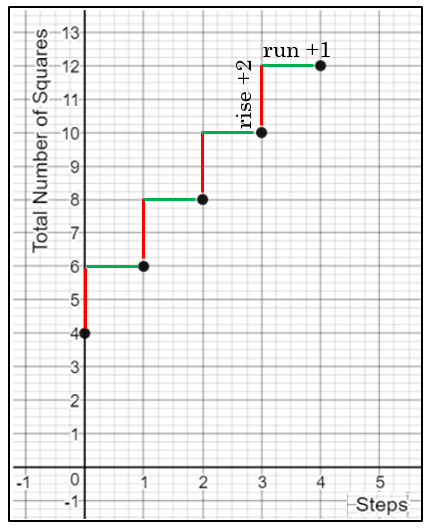# Graphed PointsThe graphed points from Pattern 1 are shown below where the $$x$$-value represents the step and the $$y$$value represents the number of squares in that step.

What do you notice about the pattern of these points?

• The points are collinear (a set of points lying in the same straight line)
• Linear relationships can be represented in tables, graphs, situations (such as a growth pattern), and equations.
• The rate of change (slope) can be identified in linear relationships.
1. Use the table from each pattern to graph the points for Patterns 2, 3, and 4 in Desmos.com.
2. Are these patterns linear relationships?
• Yes. The plotted points from Patterns 2, 3, and 4 are linear.

How can you use the graphed points to find the slope?

• Slope is the rate of change of a linear function and is a measure of the steepness of a line using the change in $$y$$ (which is the rise) and change in $$x$$ (which is the run).
• The change in a value is denoted by the symbol $$delta \Delta$$

$slope=\frac{\text {change in } y}{\text{change in }x}=\frac{\Delta y}{\Delta x} =\frac {rise}{run}$

• The slope can be found in graphed linear relationships by determining the rise and the run between any two collinear points.
• The rise and the run for Pattern 1 is shown on the graph below. Graphs are created using Desmos.com.$slope=\frac{\Delta y}{\Delta x} =\frac {rise}{run}=\frac {+2}{+1}=2$

1. Compute the slope for the remaining patterns using the graphs.
• The slope from Pattern 2 is $$\frac{2}{1}=2$$.
• The slope from Pattern 3 is $$\frac{3}{1}=3$$.
• The slope from Pattern 4 is $$\frac{3}{1}=3$$.